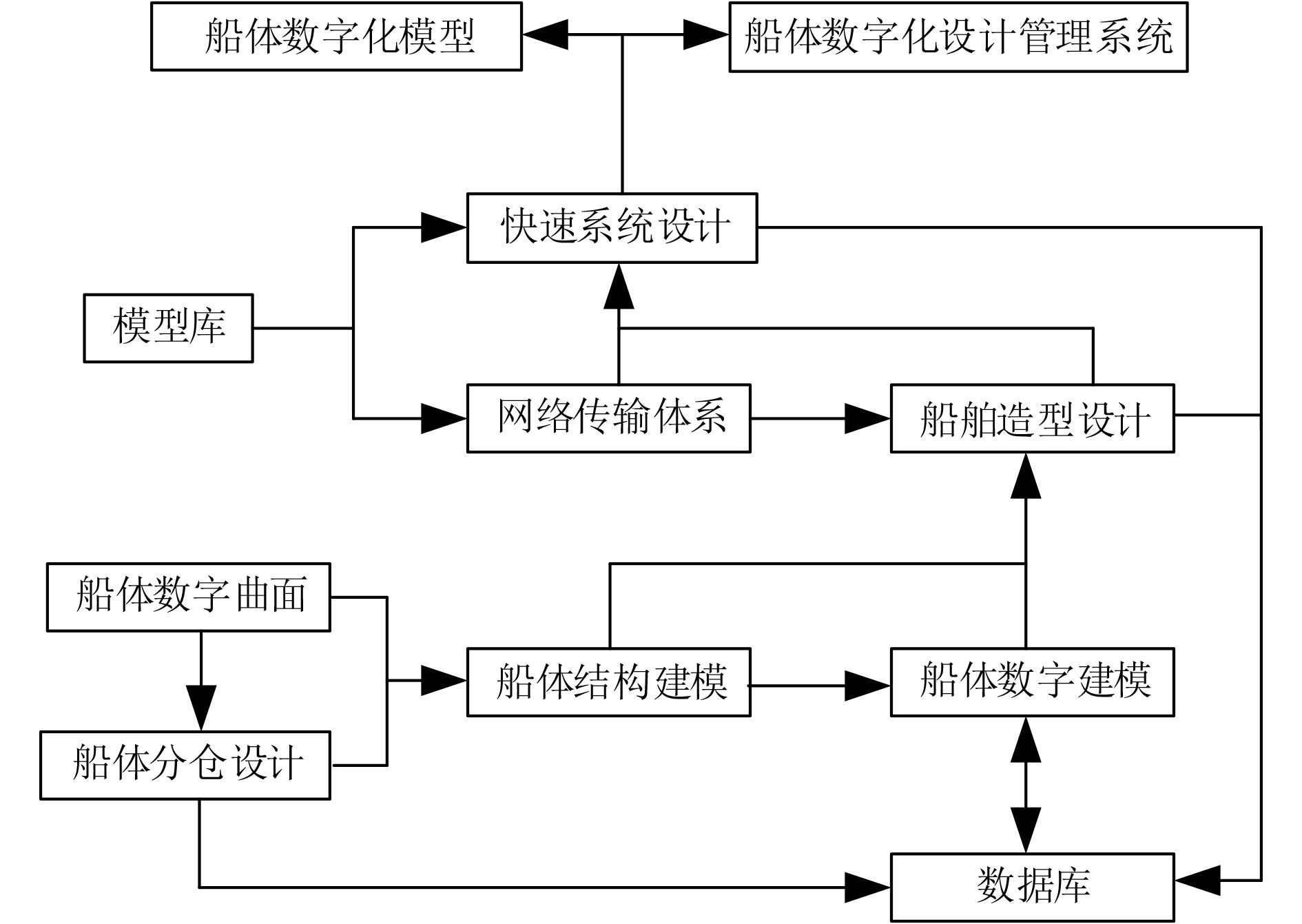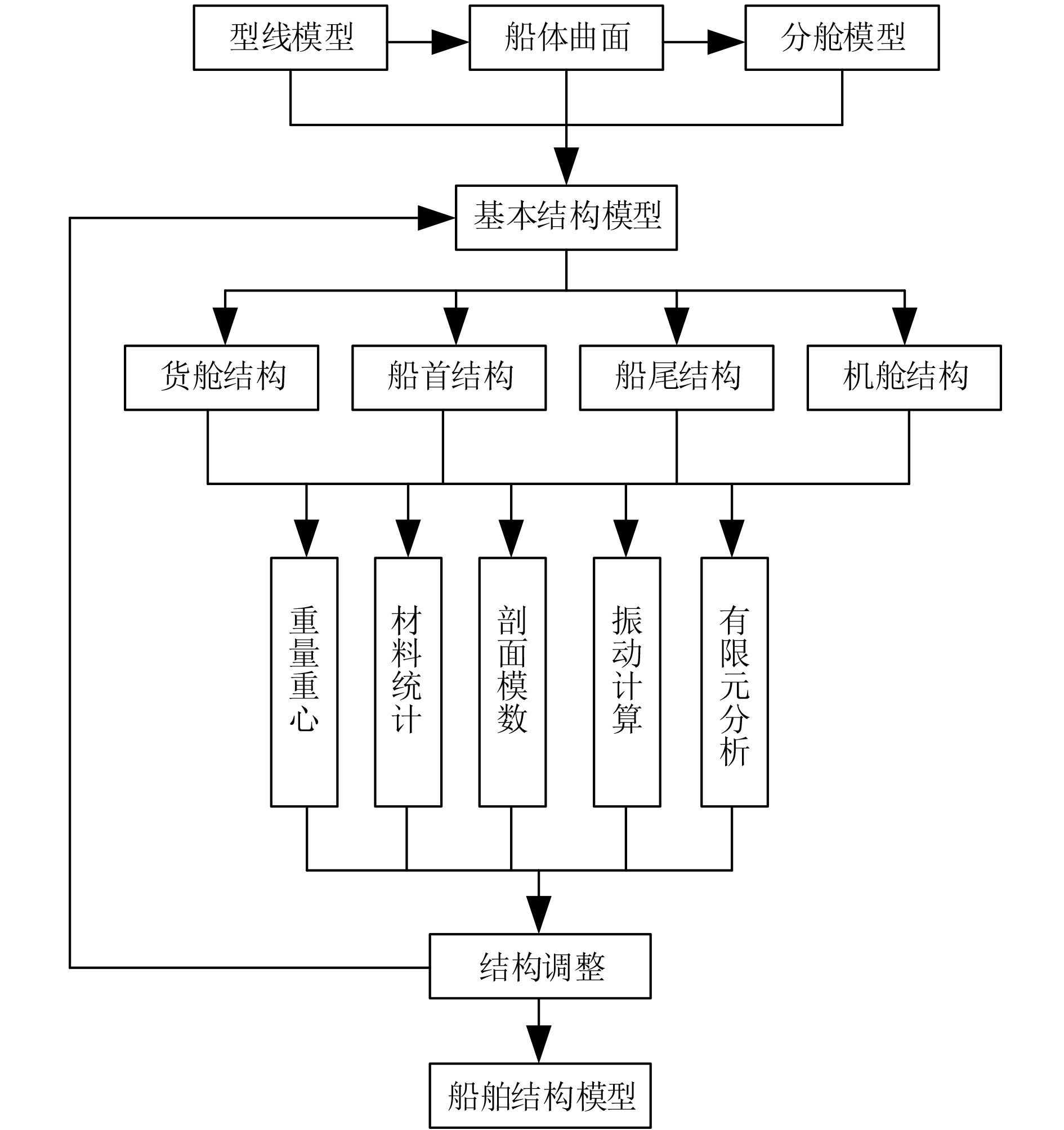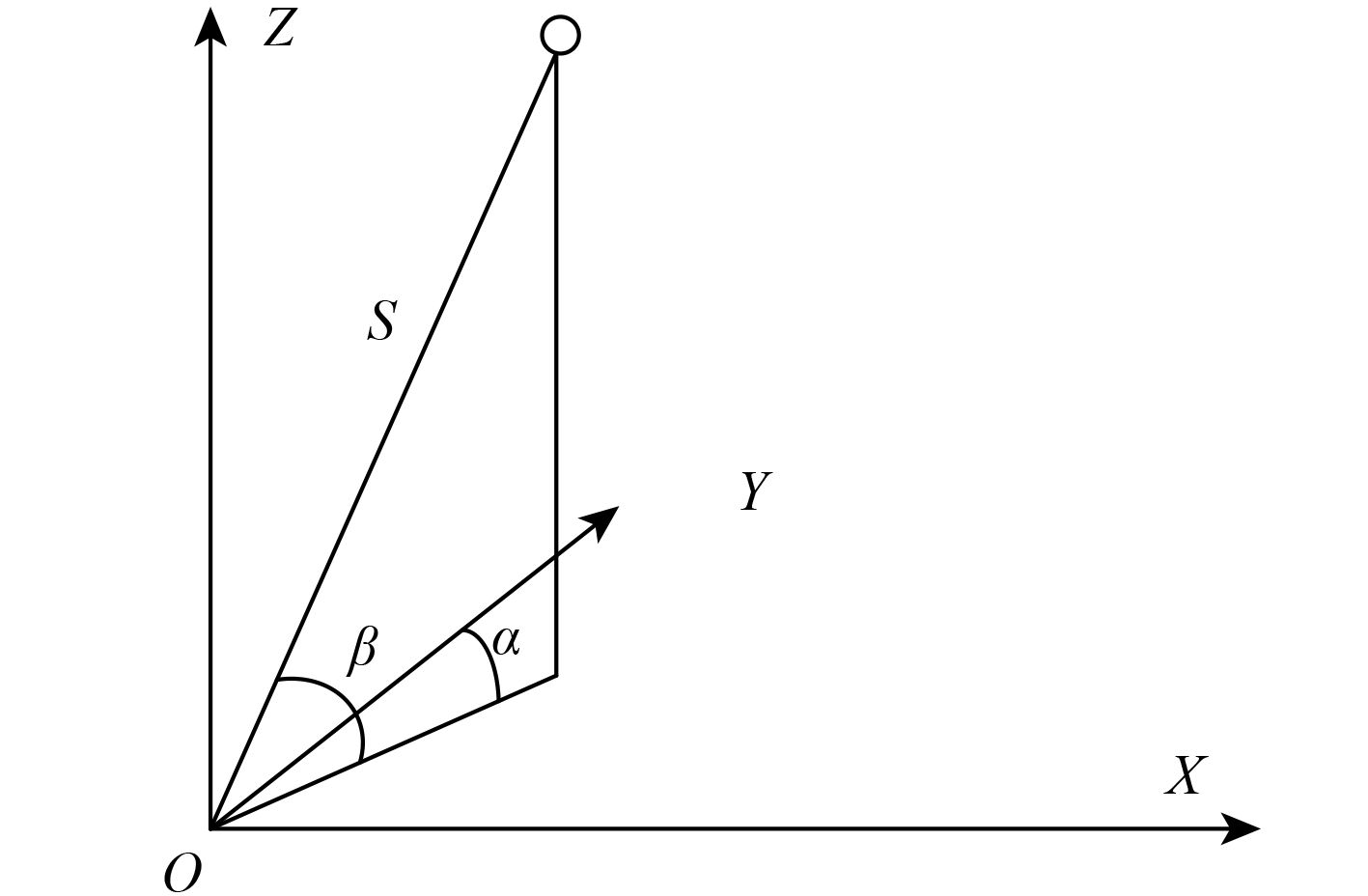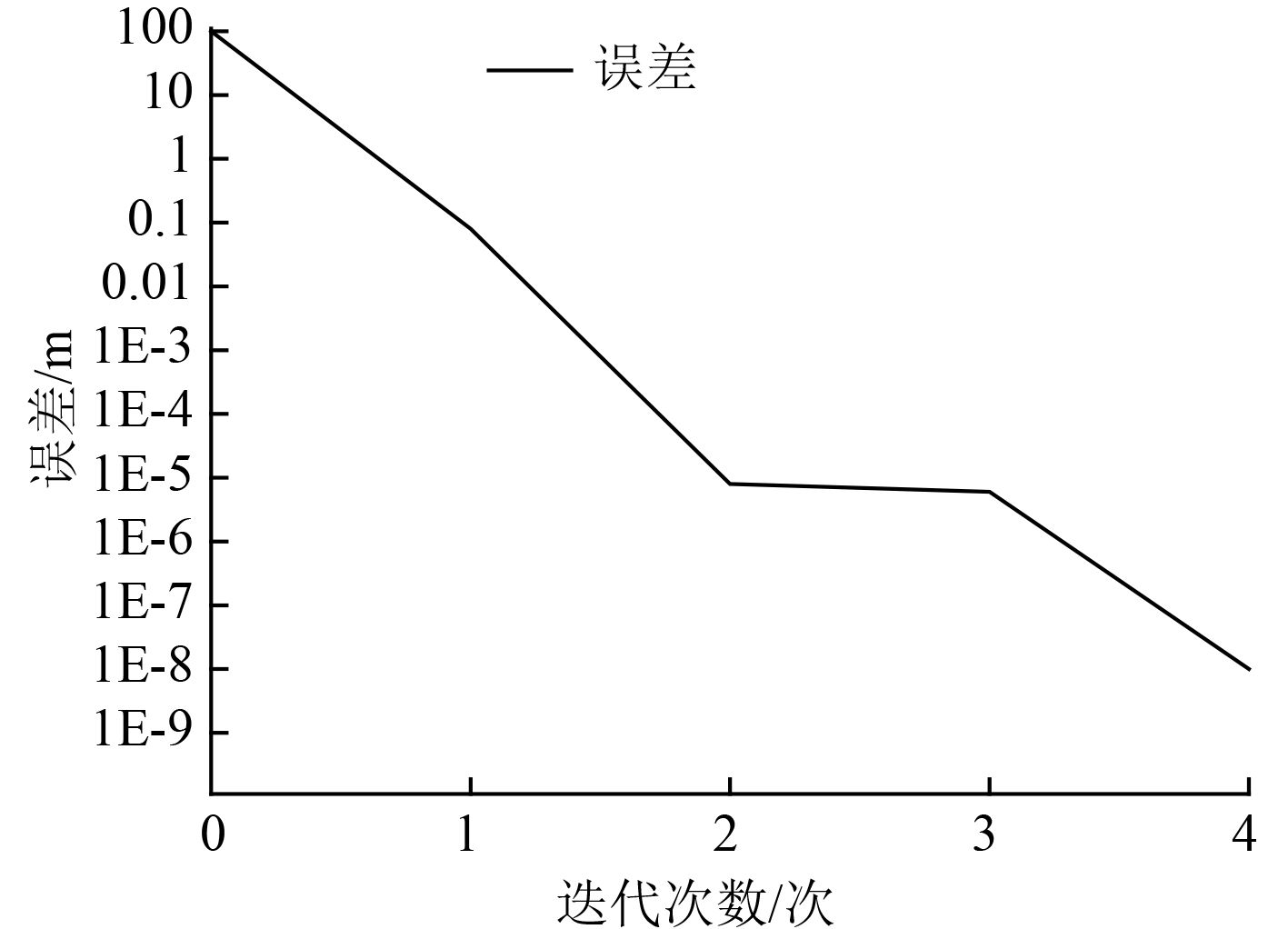﻿ 船舶数字化设计技术和分段测量数据匹配计算方法
 舰船科学技术2022, Vol. 44Issue (8): 145-148    DOI: 10.3404/j.issn.1672-7649.2022.08.030PDF

A digital design technology of ship and matching calculation method of sectional measurement data
XU Yu-chun, ZHANG Xiu-ying
Henan Wisdom Education and Intelligent Technology Application Engineering Technology Research Center, Zhengzhou 451460, China
Abstract: The test principle of total station summarizes the digital design technology of ship, and focuses on the construction of ship digital design platform, including ship digital modeling, ship structure modeling, ship rapid design and so on. The digital modeling method of ship and the modeling method of ship structure are proposed; This paper studies the ship sectional measurement data matching technology, expounds the ship sectional structure measurement technology, and finally optimizes the ship data matching technology based on ICP algorithm. The research of this paper plays the role in promoting the development of ship design and manufacturing technology.
Key words: ship     digital design     sectional measurement     matching data     algorithm
0 引　言

1 船舶的数字化设计技术 1.1 船舶数字化设计平台图 1 船舶数字化设计平台 Fig. 1 Ship digital design platform

1.2 船舶数字建模设计

 $p\left( u \right) = \sum\limits_{i = 0}^n {{p_i}{R_{i,k}}\left( u \right)} 。$ (1)

 ${R_{i,k}}\left( u \right) = \frac{{{W_i}{N_{i,k}}\left( u \right)}}{{\displaystyle\sum\limits_{j = 0}^n {{W_j}{N_{j,k}}\left( u \right)} }}\text{。}$ (2)

 $p\left( u \right) = \frac{{\displaystyle\sum\limits_{i = 0}^n {\displaystyle\sum\limits_{j = 0}^m {{W_{i,j}}{P_{i,j}}{N_{i,k}}\left( u \right){N_{j,l}}\left( v \right)} } }}{{\displaystyle\sum\limits_{i = 0}^n {\displaystyle\sum\limits_{j = 0}^m {{W_{i,j}}{N_{i,k}}\left( u \right){N_{j,l}}\left( v \right)} } }}\text{。}$ (3)

 $k = \frac{{2\sin \alpha }}{l}\text{。}$ (4)

 $C = \left( {T,01,02,V} \right)\text{。}$ (5)

1.3 船舶结构建模方法图 2 船舶结构建模方法 Fig. 2 Ship structure modeling method
2 船舶的分段测量数据匹配技术 2.1 船舶分段测量技术图 3 全站仪测试原理 Fig. 3 Test principle of total station
 $\left\{ {\begin{array}{*{20}{l}} {{X_{i,s}} = S \cdot \cos \beta \cdot \cos \alpha }，\\ {{Y_{i,s}} = S \cdot \cos \beta \cdot \sin \alpha } ，\\ {{Z_{i,s}} = S \cdot \sin \beta } 。\end{array}} \right.$ (6)

2.2 船舶数据匹配技术

ICP算法的核心在于使用全站仪测出船舶分段结构体表面的特征点数据集s，并且数据集s要包含于设计数据集m中。对船舶分段结构测量点集s中的任意坐标点进行初始变换，并在设计数据集m中找出最接近的设计点，构建出一一对应的关系，然后根据迭代ICP算法进行迭代计算，直至满足设计阈值为止。两特征点集的配准变换的目标最小函数如下式：

 $\min {\text{ }}d\left( {{R^k},{T^k}} \right) = \sum\limits_{i = 1}^N {{{\left[ {{R^k}{s_i} + {T^k} - {m_i}} \right]}^2}} \text{。}$ (7)

 $\min {\text{ }}{d^k} = \sum\limits_{i = 1}^N {{{\left[ {{R^k}{s_i} + {T^k} - {m_i}} \right]}^2}} \text{。}$ (8)

 ${e^k} = \sum\limits_{i = 1}^N {\left\| {m_i^k - s_i^k} \right\|} _2^2\text{，}$ (9)

 ${d^k} = \sum\limits_{i = 1}^N {\left\| {m_i^k - s_i^{k + 1}} \right\|} _2^2\text{，}$ (10)

 $\left\| {m_i^{k + 1} - s_i^{k + 1}} \right\|_2^2 \leqslant \left\| {m_i^k - s_i^{k + 1}} \right\|_2^2\text{。}$ (11)

ICP算法迭代次数所对应的误差如图4所示。图 4 ICP算法迭代次数和误差曲线 Fig. 4 Iteration times and error curve of ICP algorithm
3 结　语

  郝江凌, 单雄飞, 赵利宁, 等. 电子海图导航系统的研究进展与未来趋势[J]. 大连海事大学学报, 2021(47): 1-7. HAO Jiang-ling, SHAN Xiong-fei, ZHAO Li-ning, et al. Research progress and future trend of electonic chart navigation system[J]. Journal of Dalian Maritime University, 2021(47): 1-7.  甘丽霞. 基于增强现实技术的船舶智能导航系统[J]. 舰船科学技术, 2021, 43: 118-120. GAN Li-xia. Design of ship intelligent navigation system based on augmented reality technology[J]. Ship Science and Technolgoy, 2021, 43: 118-120.  龚茜茹, 赵建超. 卫星导航系统的船舶航行轨迹智能跟踪方法[J]. 舰船科学技术, 2019, 41: 37-39. GONG Qian-ru, ZHAO Jian-chao. Intelligent tracking method of ship’s navigation track information in satellite navigation system[J]. Ship Science and Technolgoy, 2019, 41: 37-39.  王刚. 船舶导航轨迹识别中智能算法研究[J]. 舰船科学技术, 2016, 38: 142-144.  李慧真. 基于大数据处理技术的界面交互设计研究[J]. 现代电子技术, 2019, 42: 38-41.  李静, 东星, 曾健友. 船舶推进监控系统终端显示界面交互设计方法[J]. 舰船科学技术, 2021, 43: 109-111. Li Jing, DONG Xing, ZENG Jian-you. Interactive design methond for terminal display interface of ship propulsion monitoring system[J]. Ship Science and Technolgoy, 2021, 43: 109-111.  刘岗, 陈超, 赵轶男, 等. 作战指挥控制系统人机交互设计流程研究[J]. 包装工程, 2020, 41: 85-91.  马超民, 赵丹华, 辛灏. 基于用户体验的智能装备人机交互界面设计[J]. 计算机集成制造系统, 2020, 26: 2650-2660.